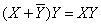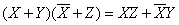Computer Organization and Structure

Homework #1

Due: 2005/10/11

1.      Assume that X consists of 3 bits, x2 x1 x0. Write four logic functions that are true if and only if

a.         X contains only one 0

b.        X contains an even number of 0s

c.         X when interpreted as an unsigned binary number is less than 4

d.        X when interpreted as a signed (two’s complement) number is negative

2.      For the above four logic functions:

a.         Construct their truth tables.

b.        What are the functions in sum of products forms, using “little m” notation?

c.         What are the functions in product of sums forms, using “big M” notation?

d.        Use the Karnaugh map method to simplify the functions in sum of products forms.

e.         Draw logic schematics using AND, OR, and INVERT gates.

3.      Prove the following simplification theorems using the first eight laws of Boolean algebra. Write the particular law or theorem you are using in each step. For each simplified function you derive, how many literals does it have?

a.b.c.d.